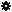﻿ Object Model: EdgeCollection.NeighborEdges

EdgeCollection.NeighborEdges

## EdgeCollection.NeighborEdgesv3.0

### Description

Returns a collection of Edge objects for all edge neighbors within a given distance.

### C# Syntax

 ```EdgeCollection EdgeCollection.NeighborEdges( Int32 in_lDistance ); ```

### Scripting Syntax

 ```oReturn = EdgeCollection.NeighborEdges( [Distance] ); ```

### Parameters

Parameter Type Description
Distance Integer Distance is an integer value representing the degree of neighborhood (eg: degree=2 for an edge means its adjacent edges plus the adjacent edges of the adjacent edges).

Default Value: 1

### Examples

#### VBScript Example

 ```set oCube = ActiveSceneRoot.AddGeometry( "Cube", "MeshSurface" ) set oGeometry = oCube.ActivePrimitive.Geometry set oVertices = oGeometry.Vertices set oNeighborEdges = oVertices(0).NeighborEdges set oNeighbors = oNeighborEdges.NeighborEdges str = "Edges neighbors of this EdgeCollection are :" for each n in oNeighbors str = str & " " & n.index next logmessage str ```